JEE  >  Newton's Law of Cooling

# Newton's Law of Cooling Notes | Study Physics For JEE - JEE

## Document Description: Newton's Law of Cooling for JEE 2022 is part of Thermal Properties of Matter for Physics For JEE preparation. The notes and questions for Newton's Law of Cooling have been prepared according to the JEE exam syllabus. Information about Newton's Law of Cooling covers topics like and Newton's Law of Cooling Example, for JEE 2022 Exam. Find important definitions, questions, notes, meanings, examples, exercises and tests below for Newton's Law of Cooling.

Introduction of Newton's Law of Cooling in English is available as part of our Physics For JEE for JEE & Newton's Law of Cooling in Hindi for Physics For JEE course. Download more important topics related with Thermal Properties of Matter, notes, lectures and mock test series for JEE Exam by signing up for free. JEE: Newton's Law of Cooling Notes | Study Physics For JEE - JEE
 1 Crore+ students have signed up on EduRev. Have you?

8. Newton's law of cooling

According to this law, if the temperature T of the body is not very different from that of the surroundings T0, then rate of cooling -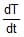is proportional to the temperature difference between them. To prove it let us assume that

T = T0 + Dt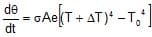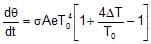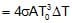if the temperature difference is small.

Thus, rate of cooling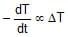or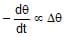as dT = dq or DT = Dq

8.1 Variation of temperature of a body according to Newton's law

Suppose a body has a temperature qi at time t = 0. It is placed in an atmosphere whose temperature is q0. We are interested in finding the temperature of the body at time t, assuming Newton's law of cooling to hold good or by assuming that the temperature difference is small. As per this law,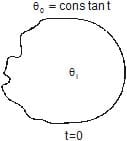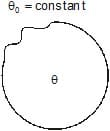rate of cooling µ temperature difference

or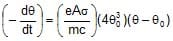or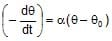Here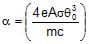is a constant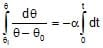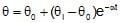From this expression we see that q = qi at t = 0 and q = qat t = ¥, i.e., temperature of the body varies exponentially with time from qi to q0 (< qi). The temperature versus time graph is a shown in figure.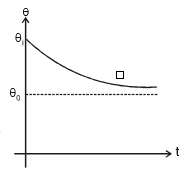Note : If the body cools by radiation from q1 to q2 in time t, then taking the approximation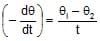and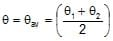The equation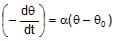becomes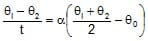This form of the law helps in solving numerical problems related to Newton's law of cooling.

8.2 Limitations of Newton's Law of Cooling :

(a) The difference in temperature between the body and surroundings must be small

(b) The loss of heat from the body should be radiation only.

(c) The temperature of surroundings must remain constant during the cooling of the body.

Ex.20 A body at temperature 40°C is kept in a surrounding of constant temperature 20°C. It is observed that its temperature falls to 35°C in 10 minutes. Find how much more time will it take for the body to attain a temperature of 30°C.

Sol.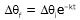for the interval in which temperature falls from 40 to 35°C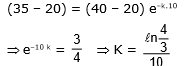for the next interval
(30 - 20) = (35 - 20) e-kt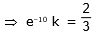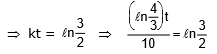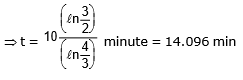Alter: (by approximate method)
for the interval in which temperature falls from 40 to 35°C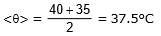from equation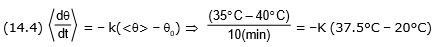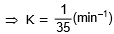for the interval in which temperature falls from 35°C to 30°C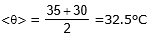from equation (14.4)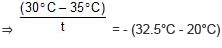⇒ required time,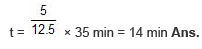9. NATURE OF THERMAL RADIATIONS : (WIEN'S DISPLACEMENT LAW)

From the energy distribution curve of black body radiation, the following conclusions can be drawn :

(a) The higher the temperature of a body, the higher is the area under the curve i.e. more amount of energy is emitted by the body at higher temperature.

(b) The energy emitted by the body at different temperatures is not uniform. For both long and short wavelengths, the energy emitted is very small.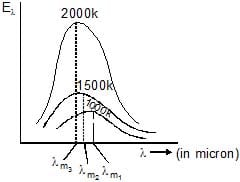(c) For a given temperature, there is a particular wavelength (lm) for which the energy emitted (El) is maximum

(d)  With an increase in the temperature of the black body, the maxima of the curves shift towards shorter wavelengths.

From the study of energy distribution of black body radiation discussed as above, it was established experimentally that the wavelength (lm) corresponding to maximum intensity of emission decreases inversely with increase in the temperature of the black body. i.e.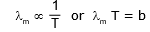This is called Wien's displacement law.

Here b = 0.282 cm-K, is the Wien's constant.

The document Newton's Law of Cooling Notes | Study Physics For JEE - JEE is a part of the JEE Course Physics For JEE.
All you need of JEE at this link: JEE

## Physics For JEE

199 videos|531 docs|256 tests
 Use Code STAYHOME200 and get INR 200 additional OFF

## Physics For JEE

199 videos|531 docs|256 tests

Track your progress, build streaks, highlight & save important lessons and more!

,

,

,

,

,

,

,

,

,

,

,

,

,

,

,

,

,

,

,

,

,

;